# Samacheer Kalvi 11th Accountancy Solutions Chapter 10 Depreciation Accounting

Students can Download Accountancy Chapter 10 Depreciation Accounting Questions and Answers, Notes Pdf, Samacheer Kalvi 11th Accountancy Book Solutions Guide Pdf helps you to revise the complete Tamilnadu State Board New Syllabus and score more marks in your examinations.

## Tamilnadu Samacheer Kalvi 11th Accountancy Solutions Chapter 10 Depreciation Accounting

### Samacheer Kalvi 11th Accountancy Depreciation Accounting Text Book Back Questions and Answers

I. Multiple Choice Questions

Question 1.
Under straight line method, the amount of depreciation is ……………..
(a) Incfeasing every year
(b) Decreasing every year
(c) Constant for all the years
(d) Fluctuating every year
(c) Constant for ail the years

Question 2.
If the total charge of depreciation and maintenance cost are considered, the method that provides a uniform charge is ……………..
(a) Straight line method
(b) Diminishing balance method
(c) Annuity method
(d) Insurance policy method
(b) Diminishing balance method

Question 3.
Under the written down value method of depreciation, the amount of depreciation is ……………..
(a) Uniform in all the years
(b) Decreasing every year
(c) Increasing every year
(d) None of the above
(b) Decreasing every yearQuestion 4.
Depreciation provided on machinery is debited to ……………..
(a) Depreciation account
(b) Machinery account
(d) Provision for depreciation account
(a) Depreciation account

Question 5.
Cash received from sale of fixed asset is credited to ……………..
(a) Profit and loss account
(b) Fixed asset account
(c) Depreciation account
(d) Bank account
(b) Fixed asset account

Question 6.
Depreciation is provided on ……………….
(a) Fixed assets
(b) Current assets
(c) Outstanding charges
(d) All assets
(a) Fixed assets

Question 7.
Depreciation is caused by ……………..
(a) Lapse of time
(b) Usage
(c) Obsolescence
(d) a, b and c
(d) a, b and c

Question 8.
Depreciation is the process of ……………..
(a) Allocation of cost of the asset to the period of its useful life
(b) Valuation of assets
(c) Maintenance of an asset in a state of efficiency
(d) Adding value to the asset
(a) Allocation of cost of the asset to the period of its useful life

Question 9.
For which of the following assets, the depletion method is adopted for writing off cost of the asset?
(a) Plant and machinery
(b) Mines and quarries
(c) Buildings
(b) Mines and quarries

Question 10.
A depreciable asset may suffer obsolescence due to ……………..
(a) Passage of time
(b) Wear and tear
(c) Technological changes
(d) None of the above
(c) Technological changesQuestion 11.
Which method shall be efficient, if repairs and maintenance cost of an asset increases as it grows older?
(a) Straight line method
(b) Reducing balance method
(c) Sinking fund method
(d) Annuity method
(b) Reducing balance method

Question 12.
Depreciation is to be calculated from the date when ……………..
(a) Asset is put to use
(d) Invoice of assets is received
(a) Asset is put to use

Question 13.
If the rate of depreciation is same, then the amount of depreciation under straight line method vis – a – vis written down value method will be
(a) Equal in all years
(b) Equal in the first year but higher in subsequent years
(c) Equal in the first year but lower in subsequent years
(d) Lower in the first year but equal in subsequent years
(b) Equal in the first year but higher in subsequent years

Question 14.
Residual value of an asset means the amount that it can fetch on sale at the of its useful life.
(a) Beginning
(b) End
(c) Middle
(d) None
(b) End

Question 1.
What is meant by depreciation?

• The process of allocation of the relevant cost of a fixed asset over its useful life is known as depreciation.
• It is an allocation of cost against the benefit derived from a fixed asset during an accounting period.

Question 2.
List out the various methods of depreciation.

1. Straight-line method or fixed installment method or Original cost method.
2. Written down value method or Diminishing balance method or Reducing balance method.
3. Sum of years digits method.
4. Machine hour rate method.
5. Depletion method.
6. Annuity method.
7. Revaluation method.
8. Sinking fund method.
9. Insurance Policy method.

Question 3.
Give the formula to find out the amount and rate of depreciation under straight-line method of depreciation.
1. Amount of depreciation per year =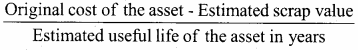2. Rate of depreciation =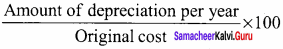Question 4.
What is the annuity method?

1. Under this method, not only the original cost of the asset but also the amount of interest on the investment is taken into account while computing depreciation.
2. The idea of considering interest is that if the investment is made in any other asset instead of the relevant fixed asset, it would have earned a certain rate of interest.
3. To calculate the amount of depreciation, the annuity factor is used. The annuity factor can be found out from the annuity table or by using a formula.
4. Amount of depreciation is computed as follows:
Amount of depreciation = Annuity factor x Original cost of the assetQuestion 5.
What is the sinking fund method?
This method is adopted especially when it is desired not merely to write off an asset but also to provide enough funds to replace an asset at the end of its working life. Under this method, the amount charged as depreciation is transferred to the depreciation fund and invested outside the business. The investment is made in safe securities which offer a certain rate of interest. Interest is received annually and reinvested every year along with the amount of annual depreciation. On the expiry of the life of the asset, the investments are sold and the sale proceeds are used for the replacement of the asset. This method of depreciation is suitable for assets of higher value. This method is also known as the depreciation fund method.

Question 1.
What are the objectives of providing depreciation?
Following are the objectives of providing depreciation:

1. To find out the true profit:

• According to the matching principle, the expenses incurred during a period must be matched with reve¬nue earned during that period.
• Hence, when an asset is used for generating income for a business, the cost of the asset attributable to the use, i.e., the reduction in the book value of the asset proportionate to the benefit derived from it, should be charged against the revenue.
• This is to be done to find out the true cost of production and profit or loss of the business for every accounting period.

2. To present the true and fair view of financial position:

• When the depreciation is charged on fixed assets, the book value of fixed assets is reduced to that extent and the remaining value is shown in the balance sheet.
• The balance represents the value of the benefit that is yet to be derived from them.
• The written down value is the true value of fixed assets which represent cost not yet written off.
• The balance sheet must represent a true and fair view of financial status.
• Hence, fixed assets must be shown at their written-down value.

3. To facilitate the replacement of fixed assets:

• When the depreciation is debited to the profit and loss account, an equal amount is either retained in the business or invested outside the business.
• When the useful life of an asset comes to an end, a new asset can be purchased by using the resources available in the business.

4. To avail tax benefits:

• As per the Indian Income Tax Act, while computing tax on business income, depreciation is deductible from income.
• Hence, depreciation is computed and charged to the profit and loss accounts to reduce tax liability.

5. To comply with legal requirements:

• Depreciation is provided on fixed assets to comply with the provisions of law apart from Income Tax Act.
• For example, Section 123(1) of the Indian Companies Act, 2013, requires every company to provide depreciation on fixed assets before declaring dividends to its shareholders.

Question 2.
What are the causes for depreciation?

1. Wear and tear
2. Efflux of time
3. Obsolescence
5. Lack of maintenance
6. Abnormal factors

Question 3.
State the advantages and limitations of the straight-line method of depreciation.
Following are the merits of the straight-line method of depreciation
(a) Simple and easy to understand – The computation of depreciation under this method is very simple and easy to understand.

(b) Equality of depreciation burden – Equal amount of depreciation is debited to the profit and loss account each year to reduce the burden of depreciation on the profit of each year is equal.

(c) Assets can be completely written off – The book value of an asset can be reduced to zero if there is no scrap value or to be the scrap value at the end of its useful life. The asset account can be completely written off.

(d) Suitable for the assets having a fixed working life – This method is appropriate for the fixed assets having a certain fixed period of working life. In such cases, the estimation of useful life is easy and in turn in helps in the easy determination of the rate of depreciation.

Limitations:
(a) Ignores the actual use of the asset – A fixed amount of depreciation is provided on each asset by applying the predetermined rate of depreciation on its original cost. But the actual use of the asset is not considered in the computation of depreciation.

(b) Ignores the interest factor – This method does not take into account the loss of interest on the amount invested in the asset.

(c) Total charge on the assets will be more when the asset becomes older – The amount of depreciation and cost of maintenance put together is less in the initial period and goes up year after year. This method did not concentrate on this.

(d) Difficulty in the determination of scrap value – It may be quite difficult to assess the true scrap value of the asset after a long period after the date of its installation.Question 4.
State the advantages and limitations of the written-down value method of depreciation.

• The equal charge against income
• Logical method

Limitations:

• Assets cannot be completely written off
• Ignores the interest factor
• Difficulty in determining the rate of depreciation
• Ignores the actual use of the asset

Question 5.
Distinguish between straight-line method and written down value method of providing depreciation.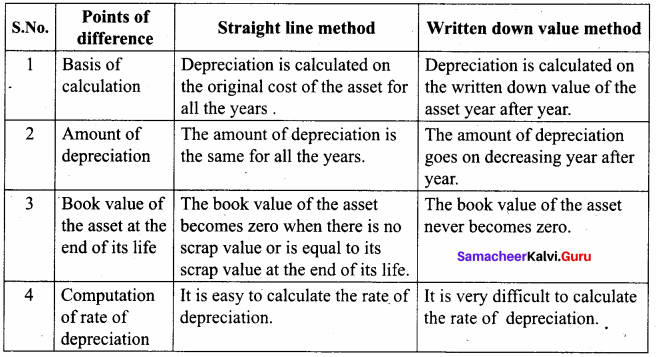IV. Exercises

Straight-line method:

Question 1.
A firm purchased a plant for ₹ 40,000. Erection charges amounted to ₹ 2,000. The effective life of the plant is 5 years. Calculate the amount of depreciation per year under the straight-line method.
Calculation of the amount of depreciation
Amount of depreciation =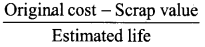Original cost = Purchase of plant + Erection charges = ₹ 40,000 + ₹ 2,000 = ₹ 42,000
Estimated life = 5 years = $$\frac{₹ 42000-0}{5 \text { years }}$$ = ₹ 8,400/-

Question 2.
A company purchased a building for ₹ 50,000. The useful life of the building is 10 years and the residual value is ₹ 2,000. Find out the amount and rate of depreciation under the straight-line method.
(1) Calculation of amount of depreciation:
Amount of depreciation =Original cost = ₹ 50,000
residual value = ₹ 2,000
Estimated life = 10 years
$$\frac{50,000-2,000}{10 \text { years }}$$ = $$\frac { 48,000 }{ 10 }$$ = ₹ 4,800/-

(2) Calculation of rate of depreciation:
rate of depreciation =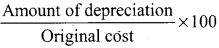= $$\frac { 48,000 }{ 50,000 }$$ x 100
= 9.6%

Question 3.
Furniture was purchased for ₹ 60,000 on 1-7-2016. It is expected to last for 5 years. Estimated scrap at the end of five years is ₹ 4,000. Find out the rate of depreciation under the straight-line method.
(1) Amount of depreciation =Original cost = ₹ 60,000
Scrap value = ₹ 4,000
Estimated life = 5 years
= $$\frac{60,000-4,000}{5 \text { years }}$$ = $$\frac { 56,000 }{ 5 }$$ = ₹ 11,200/-

Question 4.
Calculate the rate of depreciation under straight-line method from the following information: Purchased second-hand machinery on 1.1.2018 for ₹ 38,000 On 1.1.2018 spent ₹ 12,000 on its repairs. The expected useful life of the machine is 4 years Estimated residual value ₹ 6,000
Original cost – residual value
(1) Calculation of the amount of depreciation =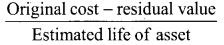Original cost = Purchase of machinery + repair charges
= 38,000 + 12,000
= 50,000
Residual value = 6,000
Estimated life = 4 years = $$\frac{50,000-6,000}{4 \text { years }}$$ = ₹ 11,000/-
(2) Rate of depreciation == $$\frac { 11,000 }{ 50,000 }$$ x 100 = 22 %

Question 5.
Calculate the rate of depreciation under the straight-line method.
Purchase price of a machine ₹ 80,000
Expenses to be capitalized ₹ 20,000
Estimated residual value ₹ 4,000
Expected useful life ₹ 4 years
Original cost – residual value =Original cost = Machine purchased + capitalised expenses
80,000 + 20,000 = 1,00,000
Residual value = 4,000
Estimated life = 4 years
= $$\frac{1,00,000-4,000}{4 \text { years }}$$ = $$\frac { 96,000 }{ 4 }$$ = ₹ 24,000/-

Question 6.
Machinery was purchased on 1st January 2015 for ₹ 4,00,000. ₹ 15,000 was spent on its erection and ₹ 10,000 on its freight charges. Depreciation is charged at 10% per annum on the straight-line method. The books are closed on 31st March each year. Calculate the amount of depreciation on machinery for the first two years.
Calculation of depreciation:
Original cost = Machinery purchased + erection charges + freight charges
= 4,00,000 + 15,000 + 10 ,000
= 4,25,000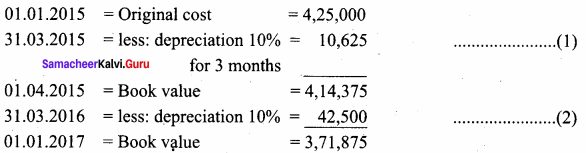First year depreciation = ₹ 10,625
Second year depreciation = ₹ 42,500

Question 7.
An asset is purchased on 1.1.2016 for ₹ 25,000. Depreciation is to be provided annually according to the straight-line method. The useful life of the asset is 10 years and its residual value is ₹ 1,000. Accounts are closed on 31st December every year. You are required to find out the rate of depreciation and give journal entries for the first two years.
Amount of depreciation =Original cost = ₹ 25,000
Residual value = ₹ 1,000
Estimated life = 10 years
= $$\frac{25,000-1,000}{10 \text { years }}$$ = $$\frac { 24,000 }{ 10 }$$ = ₹ 24,000/-

(2) Rate of depreciation == $$\frac { 2,400 }{ 25,000 }$$ x 100
= 9.6%
Journal Entries for first two years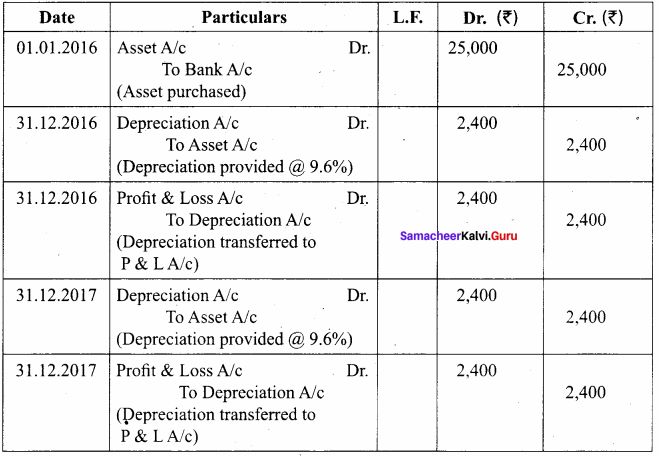Question 8.
From the following particulars, give journal entries for 2 years and prepare machinery account under straight-line method of providing depreciation:
Machinery was purchased on 1.1.2016
Price of the machine ₹ 36,000
Freight charges ₹ 2,500
Installation charges ₹ 1,500
Life of the machine 5 years
Calculation of Asset of depreciation:
Amount of depreciation =Original cost = Price of the machine + Freight charges + Installation charges
= 36,000 + 2,500 + 1,500
= ₹ 40,000
= $$\frac { 40,000 – 0 }{ 5 years }$$ = ₹ 8,000
Journal Entries for 2 years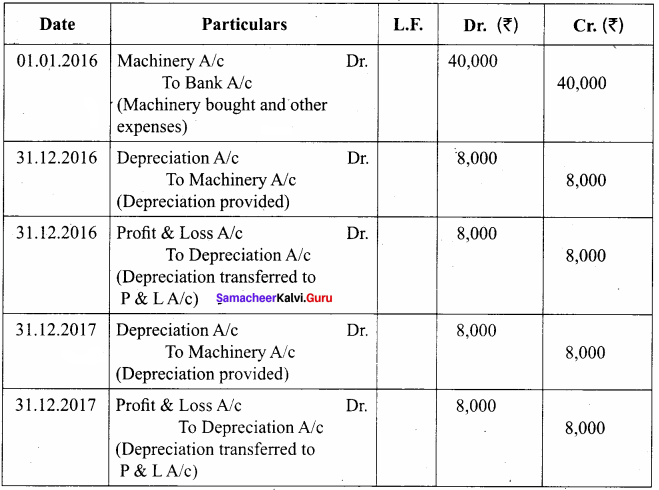Machinery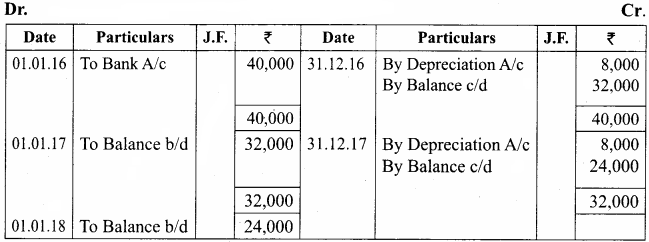Question 9.
A manufacturing company purchased on 1st April 2010, a plant and machinery for ₹ 4,50,000 and spent ₹ 50,000 on its installation. After having used it for three years, it was sold for ₹ 3,85,000. Depreciation is to be provided every year at the rate of 15% per annum on the fixed installment method. Accounts are closed on 31st March every year. Calculate profit or loss on sale of machinery.
Calculation of Profit or Loss on sale of Machinery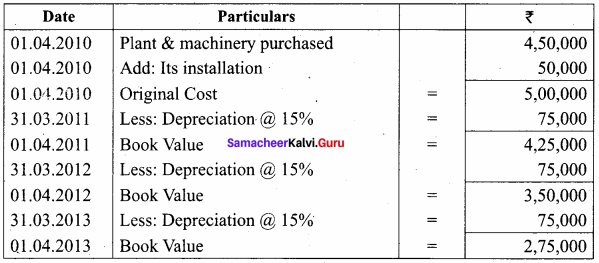Note: If the selling price is more than the book value is called profit.
Selling price – Book value = Profit 3,85,000 – 2,75,000 = 1,10,000
Profit on sale of Machinery is = ₹ 1,10,000.

Question 10.
On 1st April 2008, Sudha and Company purchased machinery for ₹ 64,000. To install the machinery expenses incurred was ₹ 28,000. Depreciate machinery 10% p.a. under the straight-line method. On 30th June 2010, the worn-out machinery was sold for ₹ 52,000. The books are closed on 31st December every year. Show machinery account.
Workings: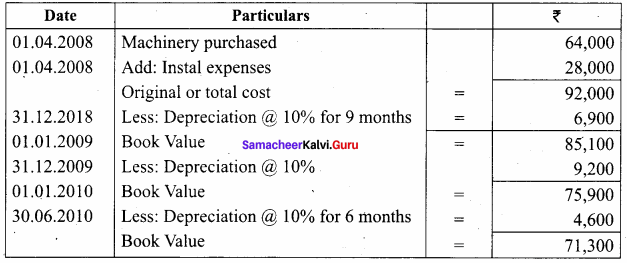If the Book value is more than the selling price it is called loss.
Book value – selling price = loss
⇒ 71,300 – 52,000 = 19,300
Loss on sale of machinery is = 19,300
Machinery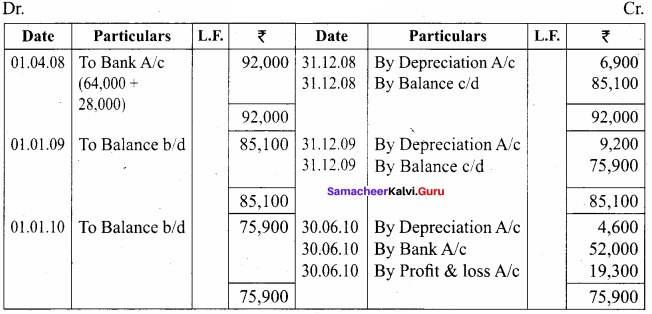Question 11.
Ragul purchased machinery on April 1, 2014, for ₹ 2,00,000. On 1st October 2015, a new machine costing ₹ 1,20,000 was purchased. On 30th September 2016, the machinery purchased on April 1, 2014, was sold for ₹ 1,20,000. Books of accounts are closed on 31st March and depreciation is to be provided at 10% p.a. on the straight-line method. Prepare machinery account and depreciation account for the years 2014 – 15 to 2016 – 17.
Workings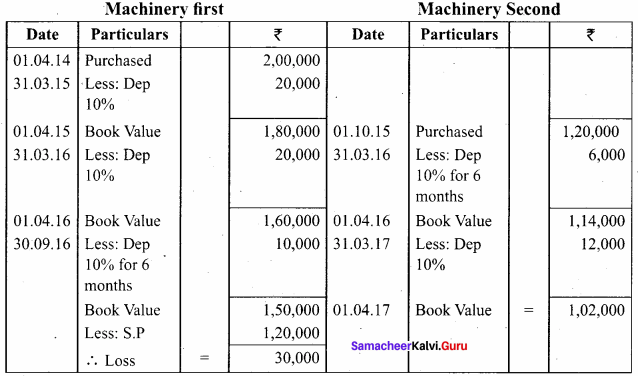Machinery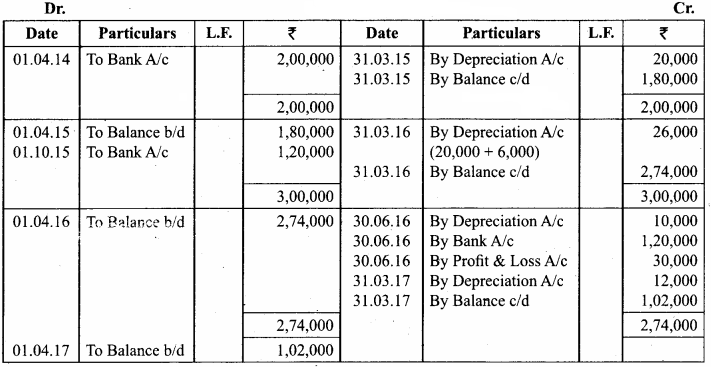Written down value method

Question 12.
An asset is purchased for ₹ 50,000. The rate of depreciation is 15% p.a. Calculate the annual depreciation for the first two years under the diminishing balance method.
Workings: Calculation of depreciation of Machinery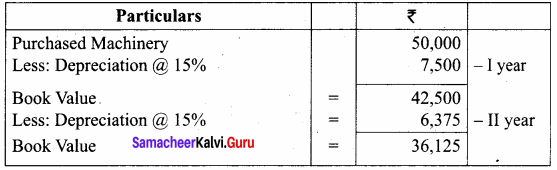Question 13.
A boiler was purchased on 1st January 2015 from abroad for ₹ 10,000. Shipping and forwarding charges amounted to ₹ 2,000. Import duty ₹ 7,000 and expenses of installation amounted to ₹ 1,000. Calculate depreciation for the first 3 years @10% p.a. on diminishing balance method assuming that the accounts are closed 31st December each year.
Calculation of the amount of depreciation on diminishing balance method: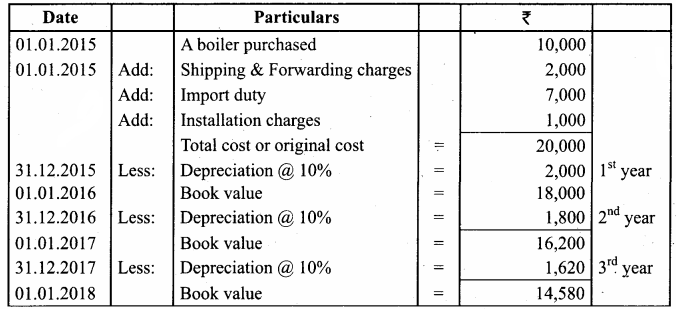2015 Depreciation ₹ 2,000
2016 Depreciation ₹ 1,800
2017 Depreciation ₹ 1,620

Question 14.
Furniture costing ₹ 5,000 was purchased on 1.1.2016, the installation charges being ₹ 1,000. The furniture is to be depreciated @ 10% p.a. on the diminishing balance method. Pass journal entries for the first two years.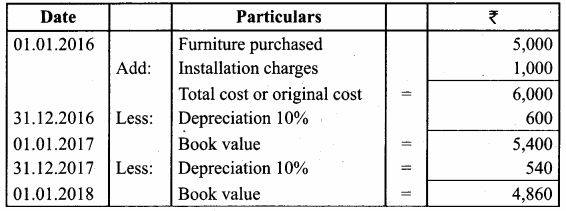Journal Entries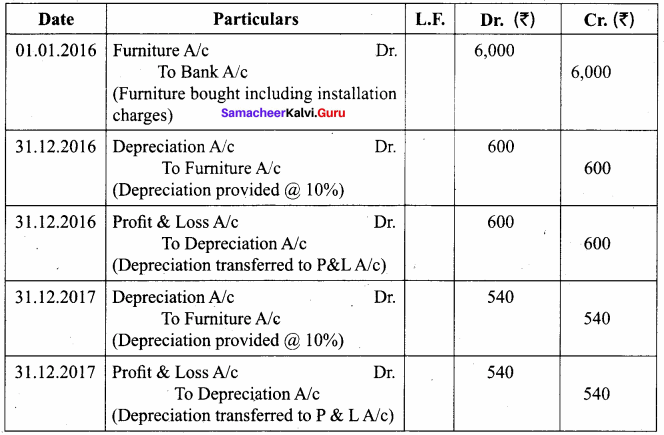Question 15.
A firm acquired a machine on 1st April 2015 at a cost of ₹ 50,000. Its life is 6 years. The firm writes off depreciation @ 30% p.a. on the diminishing balance method. The firm closes its books on 31st December every year. Show the machinery account and depreciation account for three years starting from 1st April 2015.
Workings: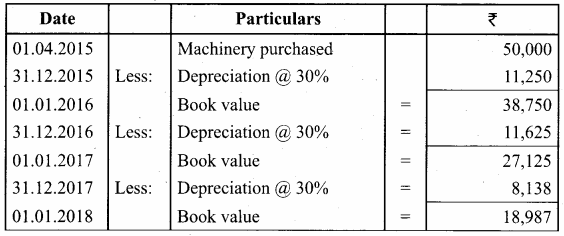Machinery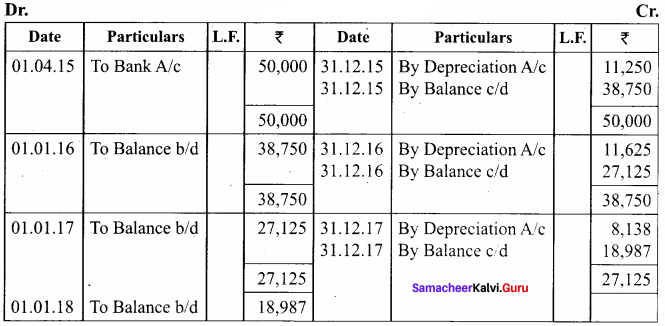Depreciation A/c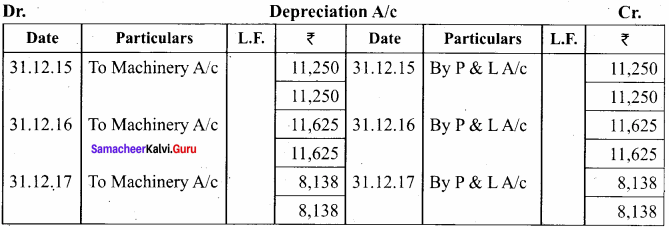Question 16.
A firm purchased a machine for ₹ 1,00,000 on 1-7-2015. Depreciation is written off at 20% on the reducing balance method. The firm closes its books on 31st December each year. Show the machinery account upto 31-12-2017.
Workings: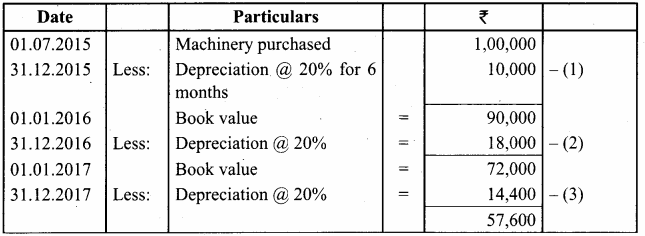MachineryA/c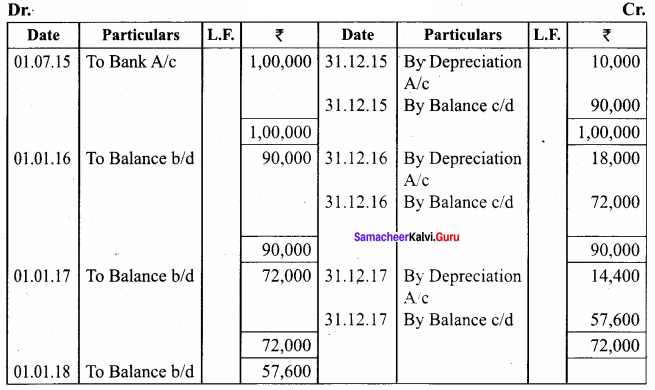Question 17.
On 1st October 2014, a truck was purchased for ₹ 8,00,000 by Laxmi Transports Ltd. Depreciation was provided @ 15% p.a. under diminishing balance method. On 31st March 2017, the above truck was sold for ₹ 5,00,000. Accounts are closed on 31st March every year. Find out the profit or loss made on the sale of the truck.
Calculation of Profit or loss on sale of the truck: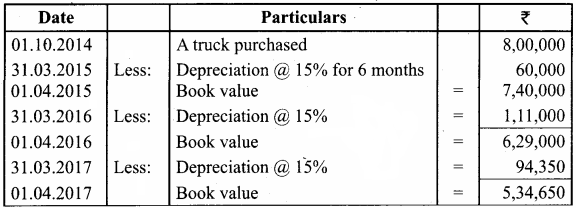Note: If Book value is more than the selling price it is called loss:
Book value – selling price = Loss
5,34,650 – 5,00,000 = 34,650
∴ Loss on sale of truck = ₹ 34,650

Question 18.
On 1st January 2015, a second-hand machine was purchased for ₹ 58,000 and ₹ 2,000 was spent on its repairs. On 1st July 2017, it was sold for ₹ 28,600. Prepare the machinery account for the years 2015 to 2017 underwritten down value method by assuming the rate of depreciation as 10% p.a. and the accounts are closed on 31st December every year.
Calculation of profit or loss on sale of machinery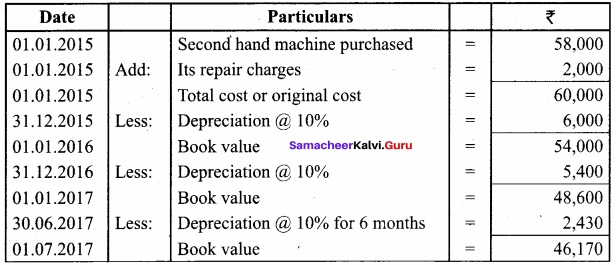Note: If Book value is more than the selling price it is called loss.
Book value – selling price = loss
46,170 – 28,600 = 17,570
∴ Loss on sale of machinery = ₹ 17,570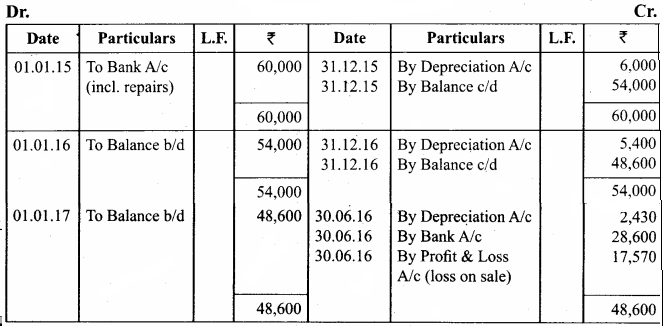Question 19.
Raj & Co purchased a machine on 1st January 2014 for ₹ 90,000. On 1st July 2014, they purchased another machine for ₹ 60,000. On 1st January 2015, they sold the machine purchased on 1st January 2014 for ₹ 40,000. It was decided that the machine be depreciated at 10% per annum on the diminishing balance method. Accounts are closed on 31st December every year. Show the machinery account for the years 2014 and 2015.
Workings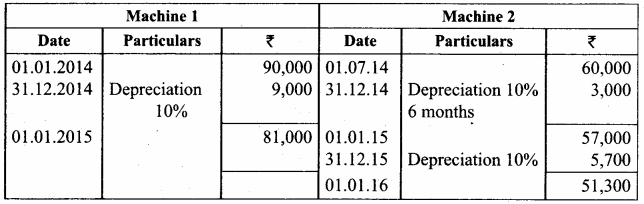Note: If Book value is more than the selling price is called loss.
Book value – selling price = loss
81,000 – 40,000 = 41,000
Machinery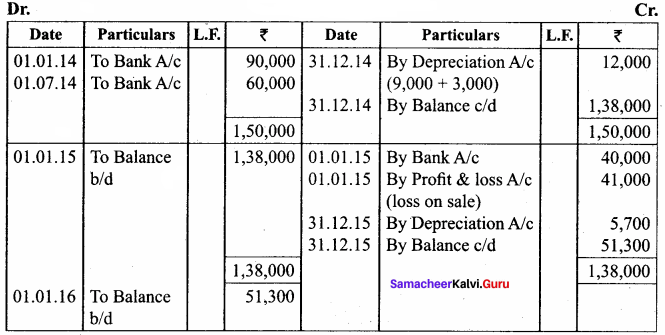Textbook Case Study Solved

Question a.
Lucky & Co’s income statement shows a loss of ₹ 3,000. The owner thinks that there is no need to provide for depreciation as the company has made a loss. He also suggests his accountant change the method of depreciation for the next year so as to avoid the loss. But, the accountant is hesitant to make the necessary changes suggested by his owner.
Now, discuss the following points:

Question 1.
Do you agree with the point that there is no need to charge depreciation when the company has made a loss?
No, I don’t agree with the point that there is no need to charge depreciation when the company has made a loss. We have to charge depreciation whether profit or loss, otherwise we cannot find out the actual profit or loss.

Question 2.
Why does the accountant hesitate to make the changes suggested by his owner?
The accountant hesitates to make the changes suggested by his owner because it will differ the profit or loss for the business. The depreciation is a necessary one, so it must be deducted every year.Question 3.
What are the accounting principles not followed if the accountant agrees to his owner’s suggestion?
If the accountant agrees to his owner’s suggestion, they will not follow the double-entry system.

Question 4.
Do you think charging depreciation could be the only reason for the company’s loss?
No, charging depreciation could not be the only reason for the company’s loss, because the business activities are subject to change but depreciation is compulsory when the business is running.

I. Multiple Choice Questions

Question 1.
Depreciation is calculated on ________ under diminishing balance method.
a) Original Cost
b) Written Down Value
c) The Scrap Value
d) None of the above
b) Written Down Value

Question 2.
Certain assets whether used or not become potentially less useful with the passage of time ……………….
(a) Efflux of time
(b) Lack of maintenance
(c) Abnormal factors
(d) Wear and tear
(a) Efflux of time

Question 3.
The process of becoming out of date or obsolete is termed as ________.
a) Depletion
b) Physical Deterioration
c) Obsolescence
d) None of the above
c) ObsolescenceQuestion 4.
Allocation of the acquisition cost of intangible fixed assets such as goodwill is called ……………….
(a) Abnormal factors
(b) Wear and tear
(c) Amortization
(d) Obsolescence
(c) Amortization

Question 5.
Meaning of Salvage value is ________.
a) Cash to be paid when the asset is disposed of
b) Estimated disposal value
c) Definite sale price of the asset
d) Cash to be received when the life of the asset ends
b) Estimated disposal value

Question 6.
The following formula is used to complete the rate of depreciation under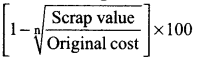……………….
(a) Written down value method
(b) Straight-line method
(c) Machine hour rate method
(d) Annuity method
(a) Written down value method

Question 1.
Define Depreciation.

1. According to Spicer and Pegler, “Depreciation is the measure of exhaustion of the effective life of an asset from any cause during a given period”.
2. According to R.N. Carter, “Depreciation is the gradual and permanent decrease in the value of an asset from any cause”.

Question 2.
What are wear and tear?
The normal use of tangible assets results in physical deterioration which is called wear and tear. When there is wear and tear, the value of the asset decreases proportionately.

Question 3.
What is ‘Obsolescence’?

1. It is a reduction in the value of assets as a result of the availability of updated alternative assets.
2. This happens due to new inventions and innovations.
3. Though the original asset is in a usable condition, it is not preferred by the users and it loses its value.
4. For example, preference of latest computers by the users.Question 4.
What is the Straight-line method?
Under this method, a fixed percentage of the original cost of the asset is charged every year by way of depreciation. Hence it is called the original cost method. As the amount of depreciation remains equal in all years over the useful life of an asset, it is also called a fixed installment method.

Question 5.
What is the Written down value of depreciation?

1. Under this method, depreciation is charged at a fixed percentage on the written down value of the asset every year. Hence, it is called the written-down value method.
2. Written down value is the book value of the asset, i.e., the original cost of the asset minus depreciation upto the previous accounting period.
3. As the amount of depreciation goes on decreasing year after year, it is called the diminishing balance method or reducing installment method.

Question 1.
What are the Characteristics of depreciation?

1. Depreciation is the process of allocation of the cost of the depreciable asset (capital expenditure) to revenue expenditure or to profit and loss account over the useful life of the asset.
2. It is the process of allocation of cost and not the process of valuation.
3. It is a decrease in the book value of the asset and not the market value of the asset.
4. It is a gradual and continuous decrease in the book value of an asset over its useful life.
5. It is calculated only for tangible depreciable fixed assets. Depreciation is not provided on intangible and wasting assets.

Question 2.
What is the machine hour rate method?